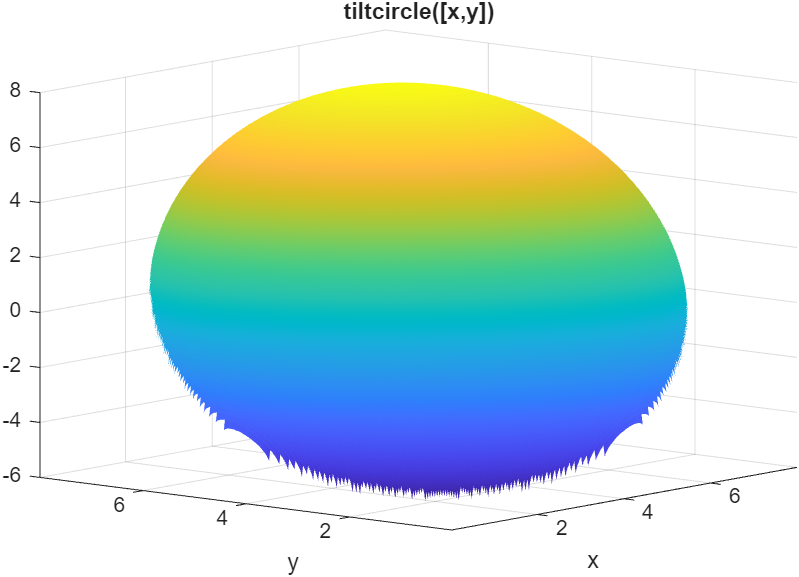Documentation

### This is machine translation

Mouseover text to see original. Click the button below to return to the English version of the page.

## Optimize Using Only Feasible Start Points

You can set the `StartPointsToRun` option so that `MultiStart` and `GlobalSearch` use only start points that satisfy inequality constraints. This option can speed your optimization, since the local solver does not have to search for a feasible region. However, the option can cause the solvers to miss some basins of attraction.

There are three settings for the `StartPointsToRun` option:

• `all` — Accepts all start points

• `bounds` — Rejects start points that do not satisfy bounds

• `bounds-ineqs` — Rejects start points that do not satisfy bounds or inequality constraints

For example, suppose your objective function is

```function y = tiltcircle(x) vx = x(:)-[4;4]; % ensure vx is in column form y = vx'*[1;1] + sqrt(16 - vx'*vx); % complex if norm(x-[4;4])>4```

`tiltcircle` returns complex values for `norm(x - [4 4]) > 4`.Write a constraint function that is positive on the set where `norm(x - [4 4]) > 4`

```function [c ceq] = myconstraint(x) ceq = []; cx = x(:) - [4;4]; % ensure x is a column vector c = cx'*cx - 16; % negative where tiltcircle(x) is real```

Set `GlobalSearch` to use only start points satisfying inequality constraints:

`gs = GlobalSearch('StartPointsToRun','bounds-ineqs');`

To complete the example, create a problem structure and run the solver:

```opts = optimoptions(@fmincon,'Algorithm','interior-point'); problem = createOptimProblem('fmincon',... 'x0',[4 4],'objective',@tiltcircle,... 'nonlcon',@myconstraint,'lb',[-10 -10],... 'ub',[10 10],'options',opts); rng(7,'twister'); % for reproducibility [x,fval,exitflag,output,solutionset] = run(gs,problem) GlobalSearch stopped because it analyzed all the trial points. All 5 local solver runs converged with a positive local solver exit flag. x = 1.1716 1.1716 fval = -5.6530 exitflag = 1 output = struct with fields: funcCount: 3254 localSolverTotal: 5 localSolverSuccess: 5 localSolverIncomplete: 0 localSolverNoSolution: 0 message: 'GlobalSearch stopped because it analyzed all the trial po...' solutionset = 1x4 GlobalOptimSolution array with properties: X Fval Exitflag Output X0```

tiltcircle With Local MinimaThe `tiltcircle` function has just one local minimum. Yet `GlobalSearch` (`fmincon`) stops at several points. Does this mean `fmincon` makes an error?

The reason that `fmincon` stops at several boundary points is subtle. The `tiltcircle` function has an infinite gradient on the boundary, as you can see from a one-dimensional calculation:

So there is a huge gradient normal to the boundary. This gradient overwhelms the small additional tilt from the linear term. As far as `fmincon` can tell, boundary points are stationary points for the constrained problem.

This behavior can arise whenever you have a function that has a square root.

Watch now